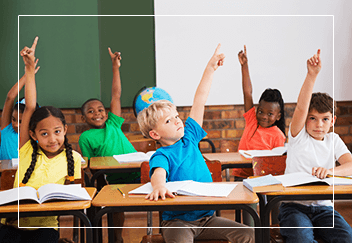# Math Tutoring

Nurturing Minds, Moulding Lives.

Step Up Academy’s Math Classes are structured around 1-on-1 instruction whether it be a small group class or individual so that our teachers can truly understand each student. We start with conceptual understanding of the topic at hand so students truly understand what it means to do math. Then, we move on to practicing and more importantly applying these math skills in different contexts. One main focus is helping our students maneuver word problems as this is a common challenge. We help our students understand math vocabulary, practice applying different concepts into various contexts, as well as building strong literacy skills.Click here to learn some tricks to overcoming the fear of mathematics.

### Number Sense and Numeration:

Focus on a general understanding of number and operations, building basic numeracy skills that can be carried forth across the strands.

Develop a solid understanding of the four basic operations which can be used to compute fluently.

Identify relationships including; part-whole- more-and-less, connections between numbers and real life quantities and measures.

Construct and understanding of numbers that allow student to make meaningful connections allowing students to apply relevant concepts, strategies and operations as they solve problems.

### Measurement

Create cross curricular connections between measurement and science, social students and physical education.

Make use of basic metric units to measure various quantities such as length, area, volume, capacity, mass and temperature.

Identify benchmarks to recognize the magnitude of units such as the metre, the litre, the kilogram.

Develop skills associated with telling time and calculating elapsed time.

Solve problems using a variety of measurement tools to develop understanding of measurement relationships.

### Geometry and Spatial Sense

Develop an intuitive awareness of surroundings and the objects in them.

Represent and describe characteristics of objects and the interrelationships in space.

Promote the use of mathematical language to support student understanding of number and measurement in geometry.

Visualize, draw and compare figures and shapes in various positions to develop strong spatial sense.

Recognize basic shapes and figures to identify geometric properties.

Become familiar with transformations of shapes including location, movement, and size.

### Patterning and Algebra

Recognize, describe and generalize patterns found in mathematical problems and real-world applications.

Build mathematical models to simulate the behavior of real-world applications that exhibit observable patterns.

Identify repeating, shrinking and growing patterns and develop strategies to extend them.

Examine how patterns change and develop an understanding of variables as changing quantities.

Represent patterns graphically, algebraically and with pictures and predict the behaviour of application questions.

Develop an understanding of how to use numbers to represent equal quantities and establish techniques for solving equations developed.

### Data Management and Probability

Explore different methods of gathering, organizing and displaying data.

Differentiate between primary and secondary data and develop techniques for analyzing teh data as well as look for bias.

Determine measures of central tendency and examine the distribution of data.

Make cross curricular connections to social studies, science and physical education.

Connect skills developed in number sense such as ratios, percents, decimals and fractions to probability.

Explore probability and data management in real-world problems that make learning relevant to students.